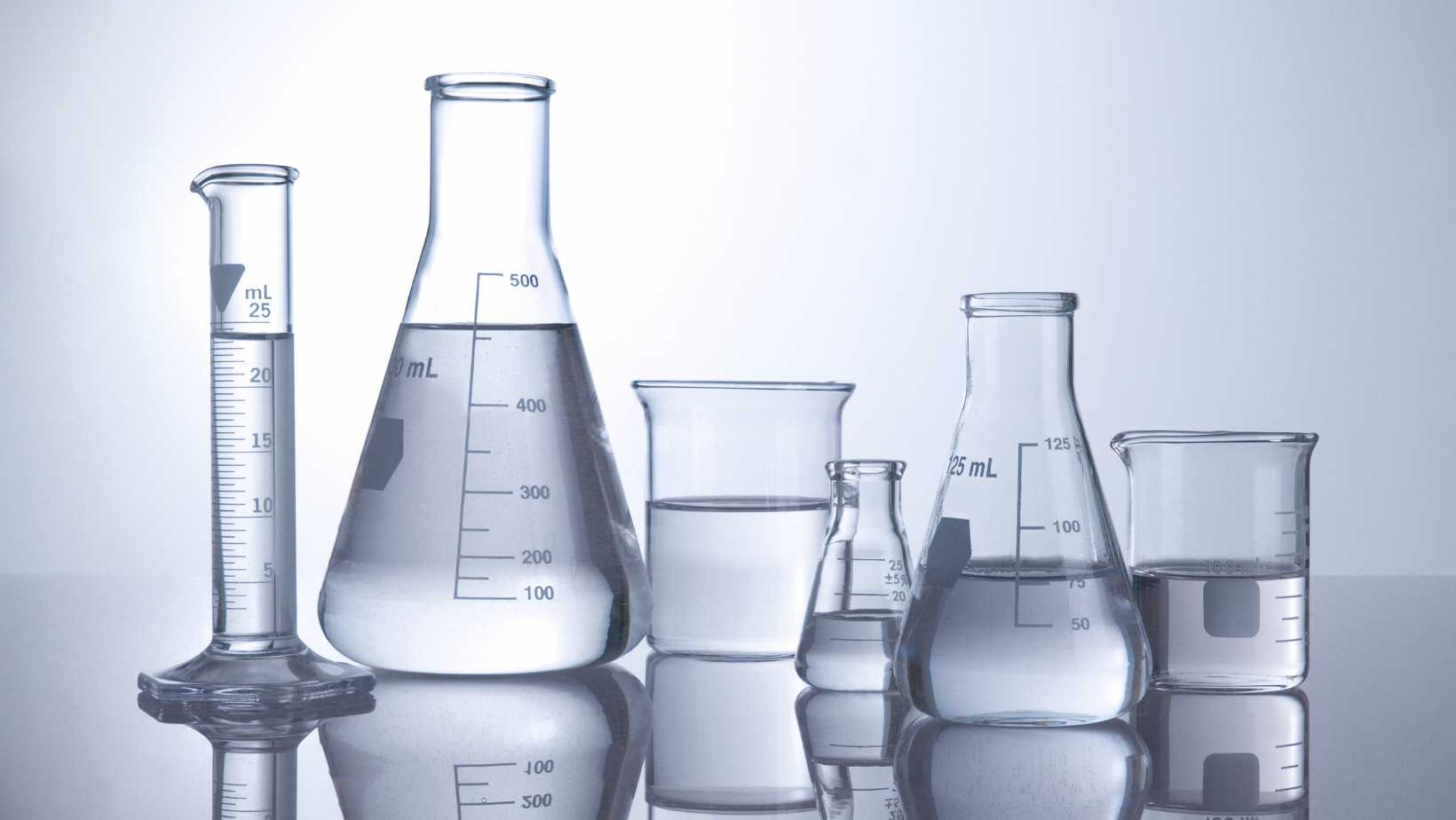# How Many ML of Water in a Gallon: Find Out The Exact ConversionCurious about how many milliliters of water are in a gallon? Well, you’ve come to the right place! In this article, I’ll provide you with a straightforward answer to this common question.

To be precise, there are approximately 3,785.41 milliliters (ml) of water in one US gallon. This conversion is based on the fact that one gallon is equal to 231 cubic inches or approximately 3.785 liters. So if you ever find yourself needing to measure out specific quantities of water in milliliters and have a gallon on hand, now you know exactly how much it contains!

Understanding conversions like these can be useful for various purposes, whether you’re measuring ingredients for cooking or calculating daily water intake. Knowing the equivalent ml measurement can save time and ensure accuracy in your measurements.

So next time someone asks “How many ml of water in a gallon?”, confidently respond with the knowledge that there are around 3,785.41 ml in one US gallon.

## How Many ML of Water in a Gallon

### Converting Gallons to Milliliters: A Basic Overview

When it comes to understanding the conversion of gallons to milliliters, we often find ourselves wondering just how many milliliters are in a gallon. Well, fret not! I’m here to shed some light on this conversion and make it easier for you.

To start off, let’s get familiar with the basic concept. A gallon is a unit of measurement commonly used in the United States to quantify volume, especially when dealing with liquids like water. On the other hand, milliliters are a metric unit of measurement used worldwide for small amounts of liquid. So when we talk about converting gallons to milliliters, we’re essentially trying to express a larger volume in terms of smaller units.

### The Conversion Formula: How to Calculate Milliliters from Gallons

Now that we have an idea of what gallons and milliliters represent, let’s dive into the conversion formula. To convert gallons to milliliters, you can use the following equation:

1 gallon = 3,785.41 milliliters

This means that there are approximately 3,785.41 milliliters in one gallon. You can think of this as multiplying the number of gallons by 3,785.41 to obtain the equivalent amount in milliliters.

For example:

• If you have 2 gallons of water, you would multiply 2 by 3,785.41 and get approximately 7,570.82 milliliters.
• Similarly, if you wanted to convert half a gallon (0.5) into milliliters using our formula above: 0.5 gallon x 3,785.41 = approximately 1,892.71 ml

Keep in mind that these values are approximate due to rounding.

### Common Conversions: Examples of Gallons to Milliliters

To further illustrate the conversion from gallons to milliliters, here are a few common examples:

• 1 gallon = 3,785.41 milliliters
• 2 gallons = 7,570.82 milliliters
• 3 gallons = 11,356.23 milliliters
• 4 gallons = 15,141.64 milliliters

As you can see, as the number of gallons increases, so does the equivalent amount in milliliters.

## Converting Gallons to Liters

When it comes to converting gallons to liters, the process is quite straightforward. Before we dive into the conversion itself, let’s briefly cover the basic units of measurement involved.

A gallon is a unit of volume commonly used in the United States and a few other countries. It is symbolized as “gal” or “g,” and there are different types of gallons, such as the US gallon and the UK gallon. For simplicity, we’ll focus on the most widely used US gallon, which equals approximately 3.78541 liters.

On the other hand, a liter is a metric unit of volume used worldwide. It is symbolized as “L” or sometimes lowercase “l.” One liter is equivalent to 0.264172 gallons.

To convert from gallons to liters, you can use a simple conversion factor: 1 US gallon = 3.78541 liters.

Here’s an example showing how this conversion works:

• Let’s say you have 5 gallons of water.
• To determine how many liters that would be, you can multiply the number of gallons (5) by the conversion factor (3.78541):
5 gal * 3.78541 L/gal = 18.92705 L
Therefore, 5 gallons would be approximately equal to 18.93 liters.

It’s important to note that this calculation provides an approximate value due to rounding decimals for easier practical use.

In conclusion, when converting gallons to liters, simply multiply the number of gallons by 3.78541 (the conversion factor). This will give you an accurate estimate of how many liters are in your given amount of water or any other liquid measured in gallons.

I hope this helps clarify how many milliliters (ml) are in a gallon and how you can convert between these two units effortlessly!

Amanda is the proud owner and head cook of her very own restaurant. She loves nothing more than experimenting with new recipes in the kitchen, and her food is always a big hit with customers. Amanda takes great pride in her work, and she always puts her heart into everything she does. She's a hard-working woman who has made it on her own, and she's an inspiration to all who know her.